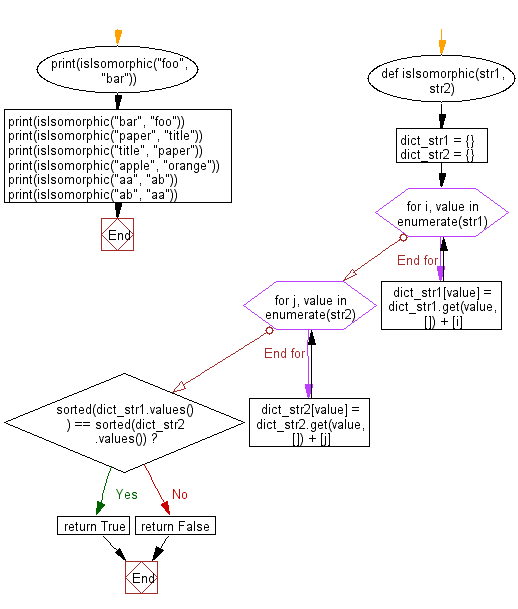﻿ Python: Check if two given strings are isomorphic to each other or not - w3resource# Python: Check if two given strings are isomorphic to each other or not

## Python Basic - 1: Exercise-69 with Solution

In abstract algebra, a group isomorphism is a function between two groups that sets up a one-to-one correspondence between the elements of the groups in a way that respects the given group operations. If there exists an isomorphism between two groups, then the groups are called isomorphic.
Two strings are isomorphic if the characters in string A can be replaced to get string B
Given "foo", "bar", return false.
Given "paper", "title", return true.
Write a Python program to check if two given strings are isomorphic to each other or not.

Sample Solution:

Python Code:

``````def isIsomorphic(str1, str2):
dict_str1 = {}
dict_str2 = {}

for i, value in enumerate(str1):
dict_str1[value] = dict_str1.get(value, []) + [i]

for j, value in enumerate(str2):
dict_str2[value] = dict_str2.get(value, []) + [j]

if sorted(dict_str1.values()) == sorted(dict_str2.values()):
return True
else:
return False

print(isIsomorphic("foo", "bar"));
print(isIsomorphic("bar", "foo"));
print(isIsomorphic("paper", "title"));
print(isIsomorphic("title", "paper"));
print(isIsomorphic("apple", "orange"));
print(isIsomorphic("aa", "ab"));
print(isIsomorphic("ab", "aa"));
``````

Sample Output:

```False
False
True
True
False
False
False
```

Pictorial Presentation:Flowchart:Python Code Editor:

Have another way to solve this solution? Contribute your code (and comments) through Disqus.

What is the difficulty level of this exercise?

Test your Programming skills with w3resource's quiz.

﻿

## Python: Tips of the Day

What is the difference between Python's list methods append and extend?

append: Appends object at the end.

```x = [1, 2, 3]
x.append([4, 5])
print (x)
```

Output:

```[1, 2, 3, [4, 5]]
```

extend: Extends list by appending elements from the iterable.

```x = [1, 2, 3]
x.extend([4, 5])
print (x)
```

Output:

```[1, 2, 3, 4, 5]
```

Ref: https://bit.ly/2AZ6ZFq